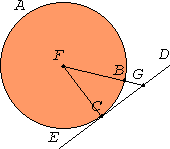Proposition 18

If a straight line touches a circle, and a straight line is joined from the center to the point of contact, the straight line so joined will be perpendicular to the tangent.
III.1

For let a straight line DE touch the circle ABC at the point C. Take the center F of the circle ABC, and join FC from F to C.I say that FC is perpendicular to DE.

I.12

For, if not, draw FG from F perpendicular to DE.

Then, since the angle FGC is right, the angle FCG is acute, and the side opposite the greater angle is greater, therefore FC is greater than FG.

But FC equals FB, therefore FB is also greater than FG, the less greater than the greater, which is impossible.

Therefore FG is not perpendicular to DE.

Similarly we can prove that neither is any other straight line except FC. Therefore FC is perpendicular to DE.

Therefore if a straight line touches a circle, and a straight line is joined from the center to the point of contact, the straight line so joined will be perpendicular to the tangent.

Q.E.D.

Guide

This proposition is used in III.19, III.36, and a other propositions in Books III and IV.